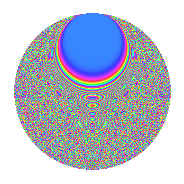# Properties

 Label 804.2.wLevel 804 Weight 2 Character orbit w Rep. character $$\chi_{804}(59,\cdot)$$ Character field $$\Q(\zeta_{22})$$ Dimension 1320 Newforms 1 Sturm bound 272 Trace bound 0

# Related objects

## Defining parameters

 Level: $$N$$ = $$804 = 2^{2} \cdot 3 \cdot 67$$ Weight: $$k$$ = $$2$$ Character orbit: $$[\chi]$$ = 804.w (of order $$22$$ and degree $$10$$) Character conductor: $$\operatorname{cond}(\chi)$$ = $$804$$ Character field: $$\Q(\zeta_{22})$$ Newforms: $$1$$ Sturm bound: $$272$$ Trace bound: $$0$$

## Dimensions

The following table gives the dimensions of various subspaces of $$M_{2}(804, [\chi])$$.

Total New Old
Modular forms 1400 1400 0
Cusp forms 1320 1320 0
Eisenstein series 80 80 0

## Trace form

 $$1320q - 18q^{4} - 5q^{6} - 22q^{9} + O(q^{10})$$ $$1320q - 18q^{4} - 5q^{6} - 22q^{9} - 26q^{10} - 15q^{12} - 44q^{13} - 34q^{16} - 13q^{18} - 66q^{21} + 74q^{22} + 13q^{24} + 80q^{25} - 110q^{28} - 36q^{30} - 22q^{33} - 54q^{34} - 37q^{36} - 72q^{37} - 22q^{40} + q^{42} - 154q^{45} - 82q^{46} + 11q^{48} + 96q^{49} - 14q^{52} - 138q^{54} - 6q^{57} + 74q^{58} + 3q^{60} - 28q^{61} - 6q^{64} - 4q^{66} - 38q^{69} - 18q^{70} - 145q^{72} + 96q^{73} + 14q^{76} - 51q^{78} - 38q^{81} - 74q^{82} - 41q^{84} - 76q^{85} + 6q^{88} - 5q^{90} + 78q^{93} - 22q^{94} + 184q^{96} - 128q^{97} + O(q^{100})$$

## Decomposition of $$S_{2}^{\mathrm{new}}(804, [\chi])$$ into irreducible Hecke orbits

Label Dim. $$A$$ Field CM Traces $q$-expansion
$$a_2$$ $$a_3$$ $$a_5$$ $$a_7$$
804.2.w.a $$1320$$ $$6.420$$ None $$0$$ $$0$$ $$0$$ $$0$$